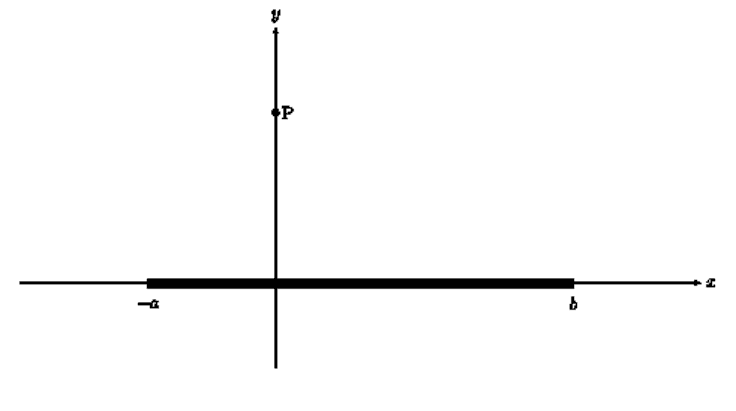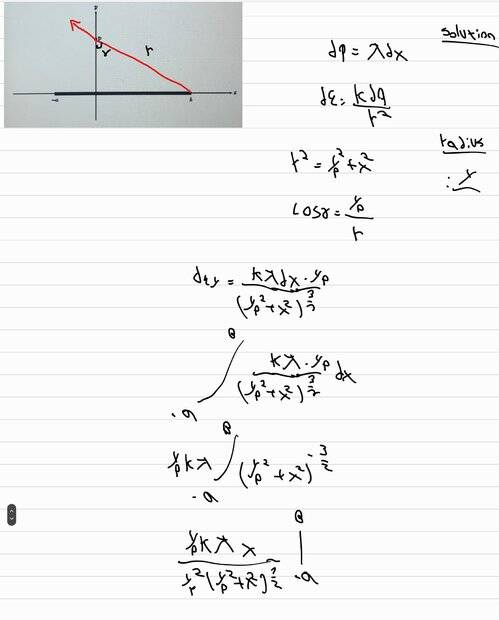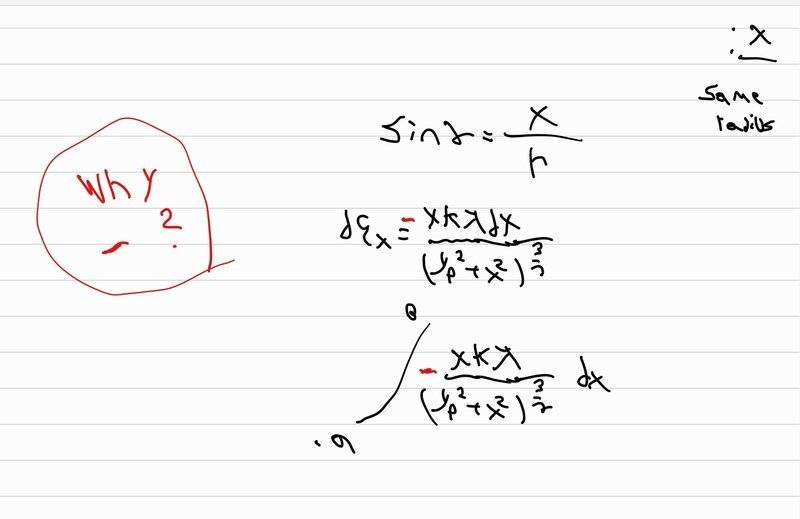# Electric field of charged rod

• yesmale4
In summary, the conversation discusses finding the correct answer for Ex and the use of minus in the solution. It is explained that the sign of the x-component depends on the sign of the linear charge density and the relative size of the bounds. The use of LaTeX in providing the solution is also encouraged.f

#### yesmale4

Homework Statement
A uniformly charged rod is positioned along the x-axis as shown in the figure. Calculate the electric field at a point P on the y-axis.
Relevant Equations
dq=lamda*dx
de=kdq/r^2
hello i would like to understand to something.
here is the drewnow for my question:
i was able to find Ey and here is my correct answer:when i try to find Ex i didnt understand something, i found the correct answer but i need to put minus before and i want to know why?
here is my solution for Ex (the correct answer):yes i know i didnt solve the integral becuase first i want to know why there is minus

There is more charge to the right of the vertical line than to the left. The sum of all the contributions from the charge on the right will be grater than the contributions from the charge on the right. So if λ is positive the overall x-component will be negative and the opposite if λ is negative.

Of course, this should be built in the answer. You say that your answer is correct, but how do you know that? It looks like the ends of the rod are at ##x=-a## and ##x=\delta,## Your final answer should have no ##x## in it; only ##a## and ##\delta## and be the sum of two expressions resulting from the integral.

Also, please use LaTeX. Your handwriting is hard to decipher at times.

when i try to find Ex i didnt understand something, i found the correct answer but i need to put minus before and i want to know why?
What is the sign of the x-component of the arrow you drew?

•vanhees71 and Chestermiller
why there is minus
Because the sign of the x component of the field due to an element dx at x has the sign of ##-\lambda x.dx##. I.e. if ##\lambda## is positive then where x is negative the field is to the right and vice versa.